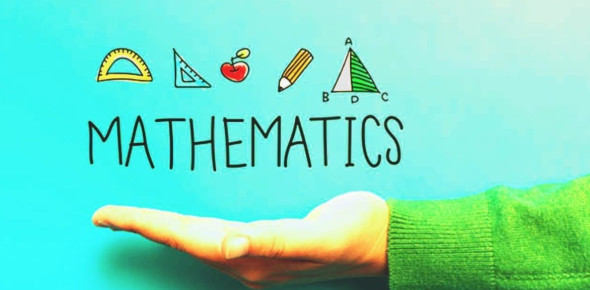# Grade 8 Mathimatics Final Exam Part 2

17 Questions | Total Attempts: 138SettingsThis test is the second part of the Math Final exam (by: pamplamousse) it is 17 questions based upon chapters 9-12 . Please answer the short answers with the correct spelling. Good luck, your halfway done :)

• 1.
Chapter 9 Linear RelationsIs this graph Linear? does this graph have a pattern?
• A.

Yes and Yes

• B.

No and Yes

• C.

No and no

• D.

Yes and No

• 2.
Which Y value is missing from the table from the equation Y=2x+1(X= -2, 0, 1, 3)
• A.

Y= -5

• B.

Y= -3

• C.

Y= 0

• D.

Y= +2

• 3.
A Linear _____________ is a line pattern that goes in a straight line
• 4.
When finding the coordinates horizontally and vertically, you are using that formula to find the ________________
• 5.
Chapter 10 Linear EquationsSolve the following by choosing the equation of this display of algebra tiles:
• A.

1x+2=1x+3

• B.

2x=5

• C.

2x=2+3

• D.

None of the above

• 6.
Find the following value of the Linear equation 4x+ 6=_____  if x=2
• A.

26

• B.

18

• C.

22

• D.

14

• 7.
Using a specific Formula, find the value of this 2 step Equation:r/10 - 3= 7     What is the value of "r"?   (Hint: use oppisite operations)
• A.

R=1

• B.

R=100

• C.

R=(-10)

• D.

R=10

• 8.
When finding the value of a two step linear equation, you use oposite operations, the first step you do to the equation is _____________ or subtract to isolate the variable
• 9.
Chapter 11 ProbabilityA ____________ is the definition of all possible outcomes in a probability experiment
• 10.
A spinner is spun, and a coin is flipped, the spinner has 4 peices of different colors, while the coin has a 1 on one side, and a 2 on the other, what is the probability of getting a yellow and a 2?
• A.

1/4x 1/2

• B.

1/4 divided by 1/2

• C.

1/6x 1/2

• D.

1/4x 1/6

• 11.
Jesse has 2 pockets, she has a loonie and a toonie in her left pocket, and a quarter, and dime and a nickle in her right pocket. What is the probability of her taking out \$1.05?
• A.

1/3

• B.

1/5

• C.

1/6

• D.

2/6

• 12.
An______________ event is the probability experiment that does not effect the rest of the outcomes
• 13.
Chapter 12 TesselationsWhich of the following can complete a tesselation plane?
• A.

Square

• B.

Octogon

• C.

Pentagon

• D.

Hexagon

• 14.
At what angle must the polygons in a tesselation must be at?
• A.

90 degrees

• B.

180 degrees

• C.

360 degrees

• D.

260 degrees

• 15.
What main shapes are used to tile the tesselation plane?
• A.

Triangles and octagons

• B.

Triangles and hexagons

• C.

Triangles and pentagons

• D.

Diamonds and pentagons

• 16.
A ______________ is the mirored image of the object rotated at a certain degree
• 17.
BONUS QUESTIONWhat is a Polygon?
Related TopicsBack to top